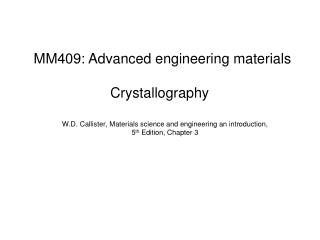DownloadDownload PresentationW.D. Callister, Materials science and engineering an introduction, 5 th Edition, Chapter 3

# W.D. Callister, Materials science and engineering an introduction, 5 th Edition, Chapter 3

Download Presentation## W.D. Callister, Materials science and engineering an introduction, 5 th Edition, Chapter 3

- - - - - - - - - - - - - - - - - - - - - - - - - - - E N D - - - - - - - - - - - - - - - - - - - - - - - - - - -
##### Presentation Transcript

1. MM409: Advanced engineering materials W.D. Callister, Materials science and engineering an introduction, 5th Edition, Chapter 3 Crystallography

2. Crystal structure • The solid materials may be classified according to the regularity with which atoms or ions are arranged with respect to one another • A crystalline materials is one in which the atoms are situated in a repeating or periodic array over large atomic distances • In crystalline structures, atoms are thought of as being solid spheres having well-defined diameters • This is termed the atomic hard sphere model in which spheres representing nearest-neighbor atoms touch one another

3. An example of the hard sphere model

4. Unit cells • The atomic order in crystalline solids indicates that small groups of atoms form a repetitive pattern. • Unit cells subdivide the structure into small repeated entities. • A unit cell is chosen to represent the symmetry of the crystal structure. • Unit cell is chosen to represent the symmetry of the crystal structure • Thus, the unit cell is the basic structural unit or building block of the crystal structure.

5. Metallic crystal structure

6. BCC

7. FCC

8. Density computations

9. Crystal systems The unit cell geometry is completely defined in terms of six parameters: 3 edge lengths, a, b and c 3 interaxial angles ,  and  These are termed as ‘lattice parameters’ of the crystal structure. Fig: A unit cell with x, y, and z coordinate axes, showing axial lengths (a, b, and c) and interaxial angles (, , and )

10. Crystallographic directions and planes When dealing with crystalline materials, it is often becomes necessary to specify some particular crystallographic plane of atoms or a crystallographic direction. 3 integers or indices are used to designate directions and planes. The basis for determining index values is the unit cell. Coordinate system consists of three (x, y and z) axes.

11. Crystallographic directions A crystallographic direction is defined as a line between two points, or a vector. Steps: • A vector of convenient length is positioned such that it passes through the origin of the coordinate system • The length of the vector projection on each of the 3 axes is determined; a, b & c • Reduce them to the smallest integer values; u, v & w • The 3 indices are enclosed in square brackets, thus: [uvw]. The , , and  directions with in a unit cell.

12. Crystallographic planes Crystallographic planes are specified by three Miller indices as (hkl). Any two planes parallel to each other are equivalent and have identical indices. A unit cell with x, y, and z coordinate axes, showing axial lengths (a, b, and c) and interaxial angles (, , and ).

13. Steps in determining (hkl) • Define origin of axis • At this point the crystallographic plane either intersects or parallels each of the 3 axes; the length of the planar intercepts for each axis is determined in terms of the lattice parameter a, b and c • Reciprocal of these numbers are taken • These numbers are changed to set of smallest integers 5. Enclose integer indices within parentheses (hkl)

14. Fig: Representations of a series each of (110) and (111) crystallographic planes.

15. Atomic arrangements Atomic arrangement depends on crystal structure Fig: (a) reduced-sphere BCC unit cell with (110) plane. (b) Atomic packing of a BCC (110) plane. Corresponding atom positions from (a) are indicated Fig: (a) Reduced-sphere FCC unit cell with (110) plane. (b) Atomic packing of an FCC (110) plane. Corresponding atom positions from (a) are indicated

16. Closed-packed crystal structures ABC, ABA, ACB, ACA

17. Figure: Close-packed plane staking sequence for hexagonal close-packed. Figure: Close-packed plane staking sequence for FCC.

18. Noncrystalline solids Fig: Two-dimensional schemes of the structure of (a) crystalline silicon dioxide and (b) noncrystalline silicon dioxide.